•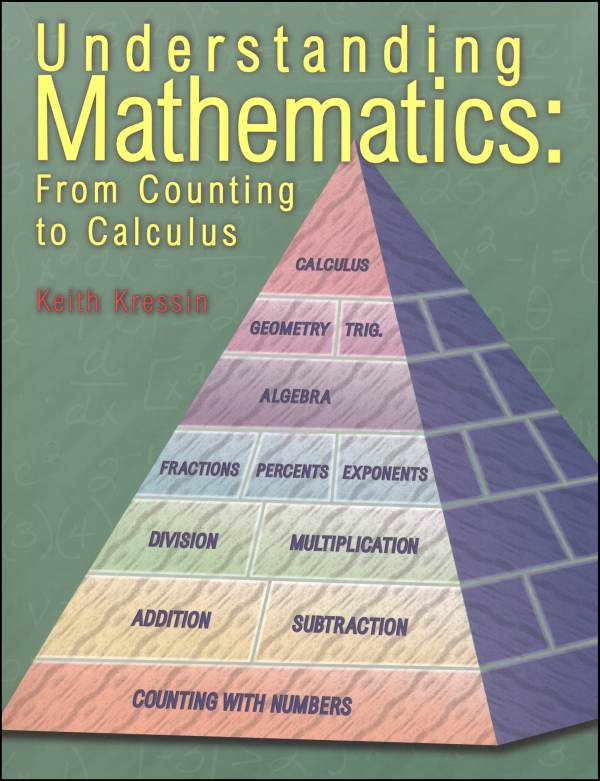•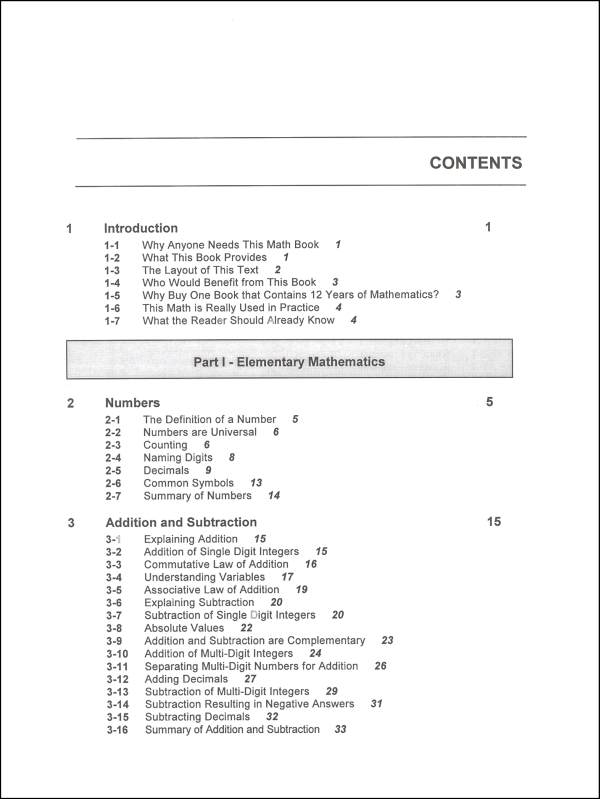•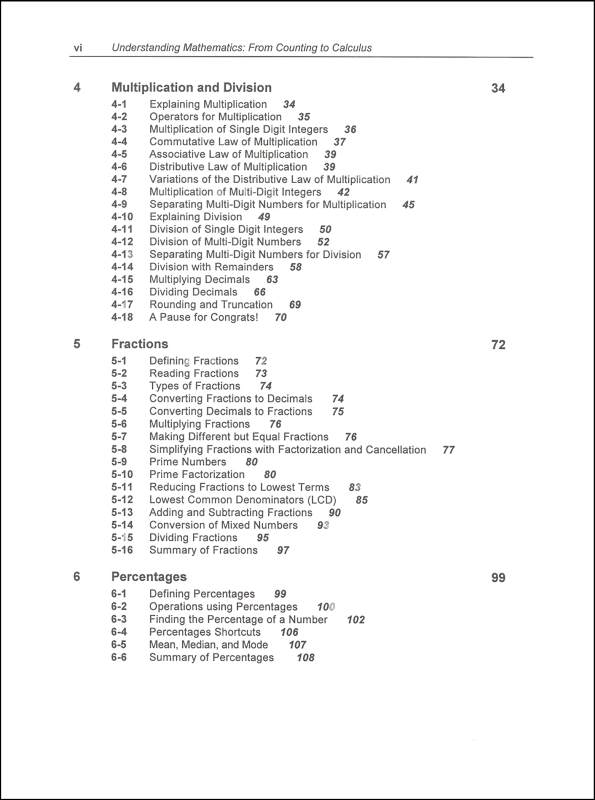•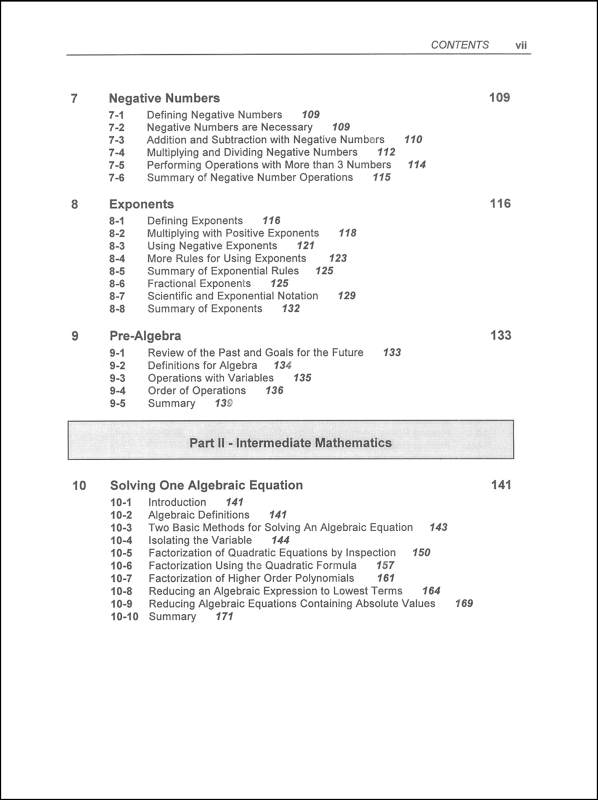•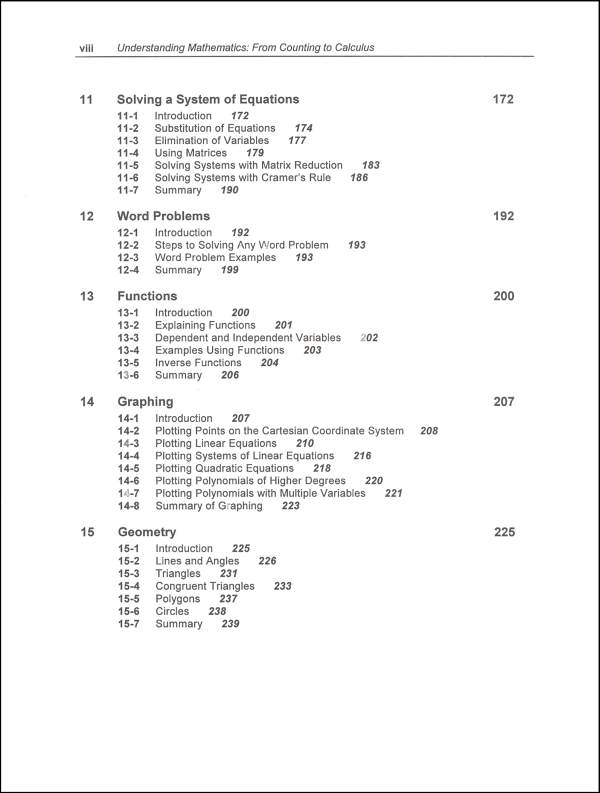•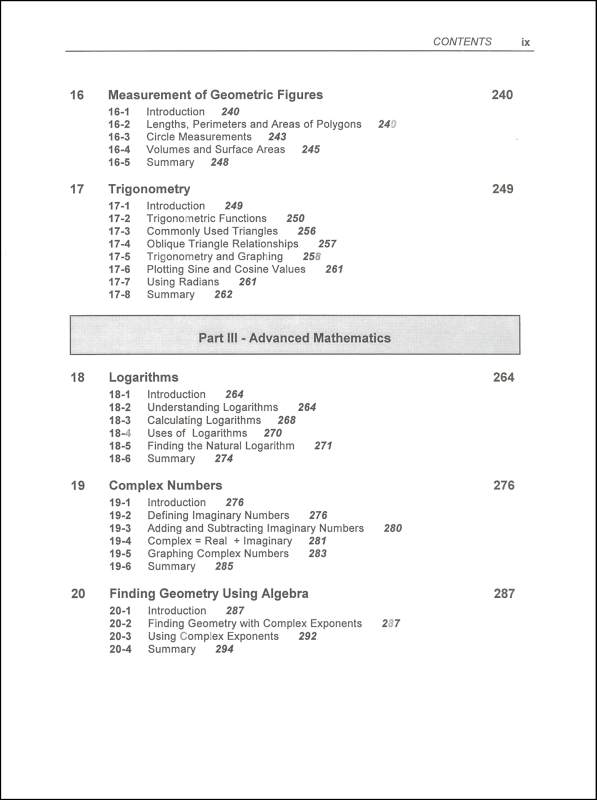•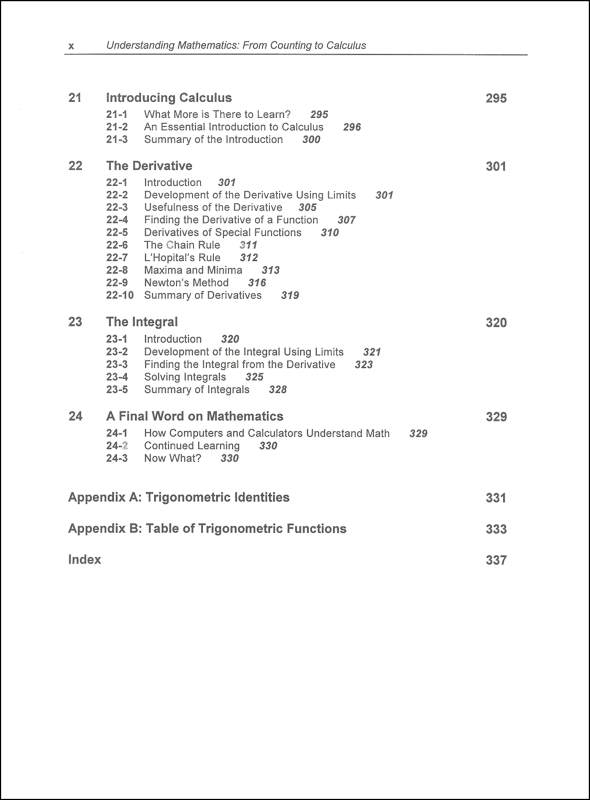•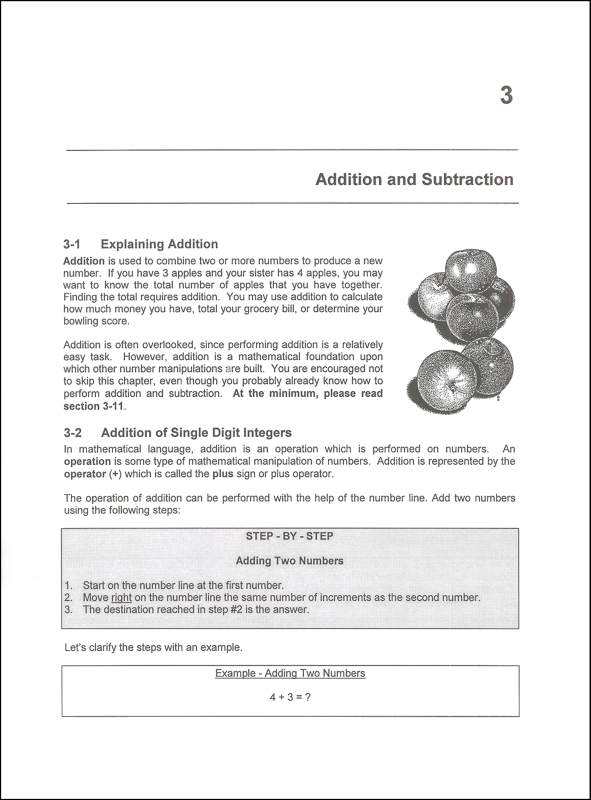# Understanding Mathematics

# 018292

Our Price: \$23.95
Retail: \$24.95
Save: 4.01% (\$1.00)
In Stock.
Qty:
Qty:

Item #: 018292 9780965730013 7-AD

#### Product Description:

A non-technical book that explains math from counting to calculus. Topics build on one another, providing the reader with a complete overview of mathematics. This book is divided into three sections - elementary (numbers, addition, subtraction, multiplication, division, fractions, percentages, negative numbers, exponents, pre-algebra), intermediate (solving one algebraic equation, solving a system of equations, word problems, functions, graphing, geometry, measurement of geometric figures, trigonometry), and advanced (logarithms, complex numbers, finding geometry, using algebra, introducing calculus, the derivative, the integral). This is not a text, but a discussion that includes numerous worked examples. For parents, students, or anyone who wants to understand math. Also useful as a comprehensive math reference.

It does no good to have a text without practice of the concepts. Students read the content found in the main text "Understanding Mathematics," then complete the corresponding section in the solutions (companion) guide. The first half of the guide offers hints, examples, explanations, exercises, and problems for practice. Each chapter's content builds on the previous chapter, and initial problems incorporate the new concept presented in the main text and more challenging problems with multiple concepts by the end of the chapter. The second half of the book gives the solutions for all of the problems in the book. A 20-question chapter summary concludes each chapter. The text along with the solutions guide provide extra practice in specific skill areas, allow students to work ahead of their curriculum for an added challenge, or can be used for a comprehensive review before taking a standardized test. Sc.~ Anh / Donna

Category Description for Understanding Mathematics:

A non-technical book that explains math from counting to calculus. Topics build on one another, providing the reader with a complete overview of mathematics. This book is divided into three sections - elementary (numbers, addition, subtraction, multiplication, division, fractions, percentages, negative numbers, exponents, pre-algebra), intermediate (solving one algebraic equation, solving a system of equations, word problems, functions, graphing, geometry, measurement of geometric figures, trigonometry), and advanced (logarithms, complex numbers, finding geometry, using algebra, introducing calculus, the derivative, the integral). This is not a text, but a discussion that includes numerous worked examples. For parents, students, or anyone who wants to understand math. Also useful as a comprehensive math reference.

It does no good to have a text without practice of the concepts. Students read the content found in the main text "Understanding Mathematics," then complete the corresponding section in the solutions (companion) guide. The first half of the guide offers hints, examples, explanations, exercises, and problems for practice. Each chapter's content builds on the previous chapter, and initial problems incorporate the new concept presented in the main text and more challenging problems with multiple concepts by the end of the chapter. The second half of the book gives the solutions for all of the problems in the book. A 20-question chapter summary concludes each chapter. The text along with the solutions guide provide extra practice in specific skill areas, allow students to work ahead of their curriculum for an added challenge, or can be used for a comprehensive review before taking a standardized test. sc.~ Anh / Donna

Primary Subject
Mathematics
7
ISBN
9780965730013
Binding
Pages
x, 342
Edition
Illustrated
Language
English
Format
Softcover Book
Brand Name
K Squared Publishing
Weight
1.85 (lbs.)
Dimensions
11.0" x 8.5" x 0.75"
Browse 2 questions Browse 2 questions and 17 answers
Why did you choose this?
Rainbow Resource Center Store
I was looking for a math reference that would cover every bit of math from elementary arithmetic processes through at least high school, and I'm hoping this fits the bill. Reviews are promising.
on Mar 19, 2021
I want to re learn mathematics.
on Dec 3, 2020
I was looking for a math reference that would cover every bit of math from elementary arithmetic processes through at least high school, and I'm hoping this fits the bill. Reviews are promising.
on Mar 19, 2021
I like the idea of a book that covers all the basics of mathematics, it's perfect for a bright kid (or adult) who can start at the beginning and work their way through.
on Jan 30, 2021
I like the idea of a book that covers all the basics of mathematics, it's perfect for a bright kid (or adult) who can start at the beginning and work their way through.
on Jan 30, 2021
This was recommended to me on a forum for Classical Conversations. I have been looking for a resource such as this for awhile, and was glad to find out it was carried on RR.
on Oct 1, 2020
What is the condition of this textbook? Understanding Mathematics
on Aug 2, 2020
BEST ANSWER: I believe all books from Rainbow are brand new. I’ve got this book at home and it it s great resource book for trouble shooting. It is also a book that can be used for all math levels from start to finish!
5.0 / 5.0
1 Review
5 Stars
4 Stars
3 Stars
2 Stars
1 Star
1
0
0
0
0
Rated 5 out of 5
I bought this some time ago and it's been sitting only to collect dust since we have NEVER used it! I like how well it's organized starting with the simple math concepts such as addition and subtraction and then working its way up to highschool math but I didn't like how the math concepts were explained
December 11, 2009

help desk software